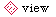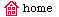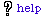## FDDS-Solver: A tool for very efficient detection of dominant strategies in normal form games

### Introduction

This tool is intended for simulation (numerical) solutions in normal-form games (see literature on Game Theory). A game in normal-form of N players is a vektor (Q;S_1,...,S_N;U_1,...,U_N), where
• Q is a set of players, i.e. Q={1,...,N}
• S_i are sets of strategies of players i\in Q, S=S_1 x S_2 x ... x S_N is a total set of profiles.
• U_i are utility functions in form U_i: S->R

By saying numerical, we mean an existence of certain computer procedure (or oracle) called cellModel, which returns a vector R^N of payoffs to each given profile s in S. When solving such a game, we have to:
• invoke cellModel(s) for all s in S,
• complete all profiles and store their utilities in memory,
• determine the equilibrium point.

Anyone can insight that this computation may be rather difficult due the fact of size of the set of profiles |S|. The algorithmic and memory complexity of the computation can be significantly decreased by transforming a given game to its strategic equivalent (it keeps all equilibrias of the original game).

Thus, we need an algorithm transforming a given game to its smaller equivalent which does not suffer from the disadvantages mentioned above. FDDS-Solver is a method and algorithm satisfying that. FDDS-Solver has been implemented as a computer tool.

### How it works

The algorithm was formally defined in .

We give a rather implementation notes here:
• FDDS-Solver is a computerized mechanism which experiment with a given game description and a cellModel
• it is assumed that the given cellModel is implemented in such a manner that each player i has just one pure best-response strategy to every sub-profile s_{-i}. This condition is not necessary, but makes the algorithm working very efficiently

### References

 Hruby, M.: Algorithmic Approaches to Game-theoretical Modeling and Simulation, In: AUCO Czech Economic Review, Vol. 2, No. 3, 2008, Praha, CZ, p. 268-300, ISSN 1802-4696, available on-line at AUCO